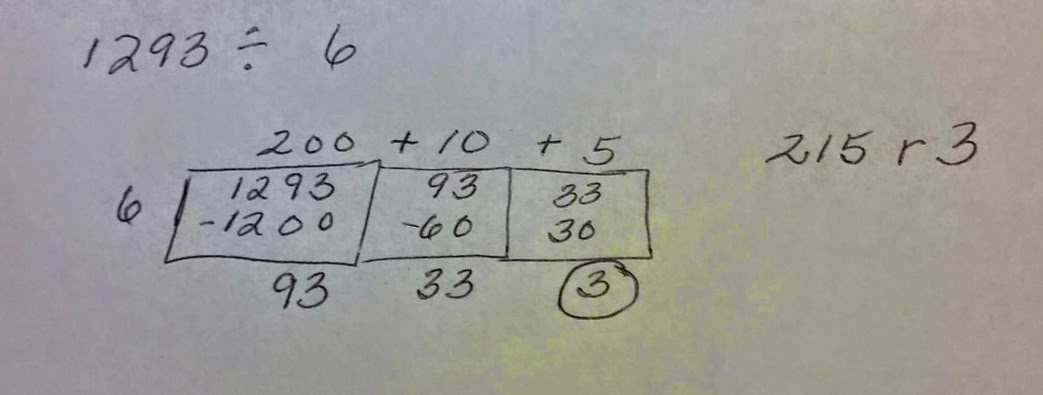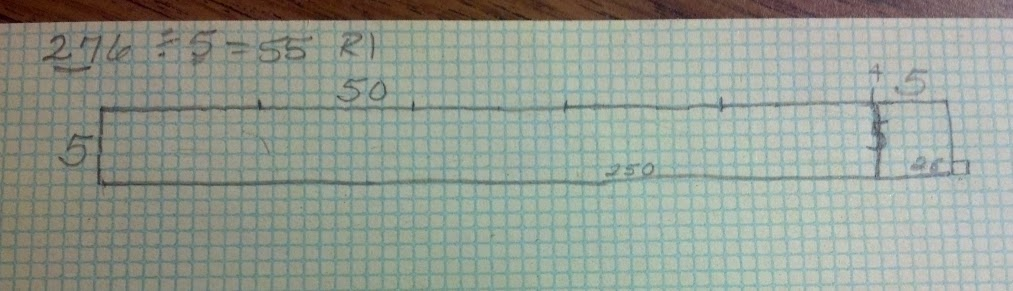# Using Area Model to Divide Thousands

27 teachers like this lesson
Print Lesson

## Objective

SWBAT divide thousands by a one digit divisor using an area model or rectangle sections method.

#### Big Idea

Students explore a place value based method to divide multi-digit dividends.

## Number Hook

5 minutes

Math magic tricks can liven up any math class and create a sense of wonder and curiosity about math. Not only that, math magic creates a new context for algebraic reasoning as students go beyond "What's the answer?" to explore "What's the trick?"

Many math magic tricks call on students to compute with the four basic operations -- sometimes applied to very large numbers. In the context of math magic, computational practice is fun. If a trick works for some students and not others, it's amazing how eager they can be to find and fix their mistakes so the trick will come out right (MP6). Best of all, many students have an inner motivation to understand how math magic tricks work, and that curiosity can lead them to embrace and apply both new and familiar concepts and skills, including algebra (MP2, MP7).

My students liked yesterday's trick so much, I did it again. I have about 3 students who are very close to figuring the math out behind this trick. Here is a description of the method for my Amazingly Cool Number Crunching Card Trick where you come up with a number of cards in a pile.

Teacher's Note: Watch and listen to this student as she explains why this trick works. She isn't able to verbalize the exact and precise reasons, but she is very close and knows "what" to do with the numbers.  She also told me that she went home and showed her parents how to do the trick and tried it several times before class today.  Amazing! That's what I want, magic to truly ignite a student's curiosity to figure out the "math behind the trick."

## Warm Up

5 minutes
Being able to multiply and divide fluently within 100 is essential during the early years of schooling, and the basic facts of addition subtraction and multiplication/division are a critical baseline, not only then but also during later work with fractions, decimals, ratio, proportion, and more.

One thing that I keep in mind when I teach this lesson is the fact that as students begin to learn math facts, their brains are focused on those basic computations, but as students become more automatic with their recall of basic facts, their brains are better able to focus on other aspects of the task like place value or fractional parts of a whole. Research conducted on how students learn mathematics has helped us to understand this important fact: being automatic with basic facts frees the brain to focus on other math processes. So, committing basic math facts to memory speeds up math tasks. As math tasks increase in complexity, they often require multiple steps to find the solution, so the importance of recall of basic facts increases.

Because basic facts are so important for my students to master, and more than half of my class does not have them mastered, I chose an oral division facts practice for this warm up. To implement this task I change the PowerPoint slide every 3 seconds. I encourage my students to yell their answers "loud and proud."

## Concept Development

40 minutes

As I teach this lesson, I keep in mind Math Practice Standard 4 - Model with Mathematics. Models can help make multiplication and division with integers more tangible. They provide a visual representation of the structure of the problem or the algorithms that can be used to perform the operations necessary to solve the problem. Models help my students see how the algorithms relate to what is actually happening in the problem.

This lesson builds from a previous lesson. My students will continue to work with an area model or a rectangle sections model to divide thousands in addition to hundreds. My area model to divide thousands video explains the method. I begin by modeling 1,293 divided by 6 on the board as students also model in their math notebooks.  You can see the completed calculation in this image:The video provides important details about the process used to get to this point and how I help my students to connect this representation to using area models in multiplication.

For the remainder of this lesson, my students work with their learning partners to practice using this strategy. We use the following process to generate expressions:

1. Students take turns drawing four cards (from a regular deck of playing cards) to create a four digit dividend.
2. Next a student draws one card to serve as the one-digit divisor
3. Learning partners work together to solve the division problems on their personal whiteboard.

I choose this approach to motivate Math Practice Standard 3 - construct viable arguments and critique the reasoning of others.  The partner practice also makes  it possible for me to circulate the room, question students, and observe them solving division problems using the area model. I can then guide and question students to deeper levels of thinking, clear up misconceptions, and provide scaffolds for students needing support, in other words differentiate the lesson as needed.

One scaffold I often provide for students is to have them use only three cards to make a number in the hundreds.  I sometimes have these students draw their area model on graph paper to they could begin to make connections between the model, arrays, and area. See photo below for an example of a student drawing 276 / 5 on graph paper to build the area model.As I interact with students I am on the lookout for interesting examples that students may be able to share with their classmates as we approach the end of the lesson. See my Modeling as Reflective Problem Solving reflection for an example of how I use my Walk and Talk strategy in this lesson.

## Student Debrief

10 minutes

Today, I used an Exit Ticket as an end of lesson formative assessment. I chose to have students solve 3248 divided by 5.

I chose to use 5 as the divisor for this task because my students know their multiplication facts for five, thus they are more easily able to build their rectangles for the model.  I wanted to make sure the Exit Ticket would assess my students' ability to use the area model, not their grasp of multiplication and division facts.  So, I chose a sufficiently challenging dividend and a friendly divisor.

I have included three videos discussing examples of student work to demonstrate my approach to assessing students using their work on an Exit Ticket: